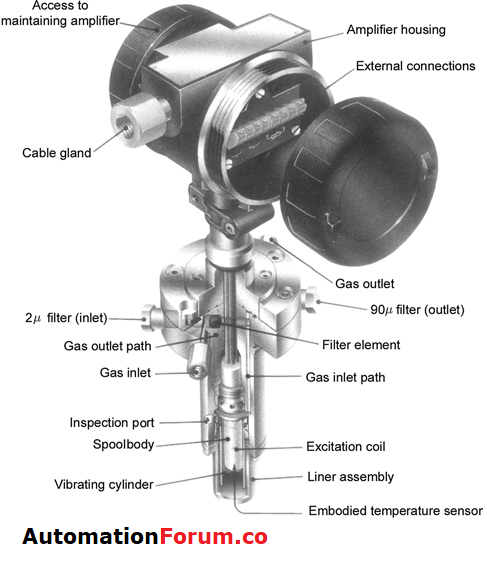# Gas Density Measurements### Principle of gas density measurement:

The relationship between temperature, pressure, and volume
of a gas is given by:

PV = nZRT

where P is the absolute pressure, V is the volume, and n is the number of moles. Z is the compressibility factor, R0 is the universal gas constant, and T is the absolute temperature.

When the specific heat is assumed to be only temperature dependent, the gas is referred to as ideal. If the ideal relative density RD of a gas is defined as the ratio of molecular weight of the gas to that of air, then

Thus, the signal from the density transducer provides an indication of the molecular weight or specific gravity of the sampled gas.

## Density transducer:

Measuring lower gas densities requires a more sensitive element. By using a thin – walled cylinder resonated in the hoop or radial mode, the Solartron 7812 gas density transducer shown in the above figure fulfills this task.

The maximum amplitude of vibration occurs with nodes at each end in the middle of the cylinder and is thus clamped at one end with a free node – forming ring at the other end.

### Working:

The cylinder is immersed in the gas whose density is to be measured and is therefore not stressed because of the gas pressure. Gas in contact with the cylinder is oscillated and effectively increases the mass of the vibrating system so that its resonant frequency is reduced.

Oscillation is electromagnetically maintained by positioning the drive and pick – up coils inside the cylinder and connecting them to a maintenance amplifier

By building the cylinder from material with a low-temperature expansion coefficient, a low-temperature coefficient is obtained. Depending on the required density range, the cylinder wall thickness varies from 0.05 to 0.15 mm, the corresponding density ranges varying from 0 to 60 kg / m3 and from 40 to 400 kg / m3.

Scroll to Top